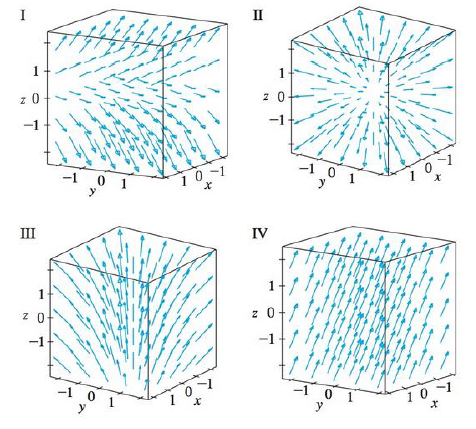Chapter 16.1, Problem 17E

Chapter
Section
Textbook Problem

Match the vector fields F on ℝ3 with the plots labeled I-IV. Give reasons for your choices.17. F(x, y, z) = x i + y j + 3 kTo determine

To match: The vector field F(x,y,z)=xi+yj+3k with the plots labeled as I-IV.

Explanation

Given data:

F(x,y,z)=xi+yj+3k=x,y,3

Formula used:

Consider a three-dimensional vector F=x,y,z .

Write the expression for length of the three dimensional vector.

|F(x,y,z)|=x2+y2+z2 (1)

Find the length of F(x,y,z) using equation (1)

Still sussing out bartleby?

Check out a sample textbook solution.

See a sample solution

The Solution to Your Study Problems

Bartleby provides explanations to thousands of textbook problems written by our experts, many with advanced degrees!

Get Started

61/2

Applied Calculus for the Managerial, Life, and Social Sciences: A Brief Approach

Change 3yd2tom2.

Elementary Technical Mathematics

30. If a variable x is normally distributed, with and

Mathematical Applications for the Management, Life, and Social Sciences

Solve for x: e2x1 = 10. a) 12(1+10e) b) 3 + ln 10 c) 2 + ln 10 d) 1+ln102

Study Guide for Stewart's Single Variable Calculus: Early Transcendentals, 8th